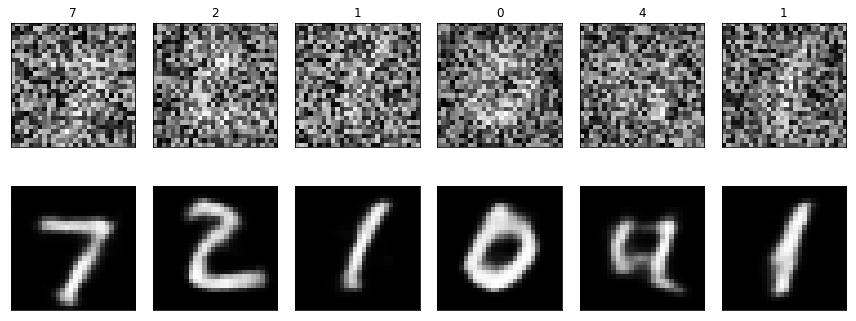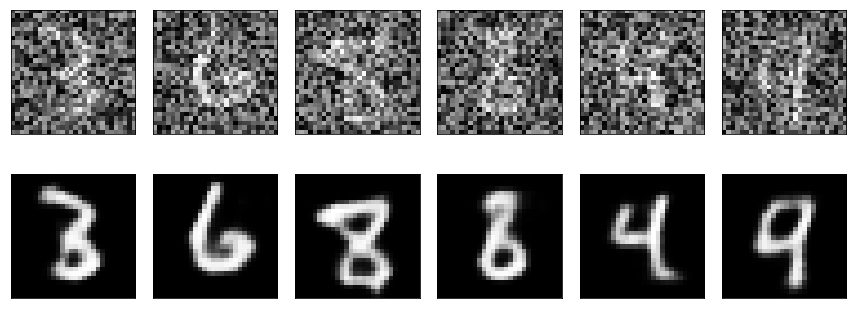## Autoencoders

An autoencoder is a NN structure used to extract a determined number of important feature from data, using unsupervised learning. The idea is to learn an efficient representation of the data (i.e. encoding) which has a much smaller dimension when compared with the original data itself. The working principle is simple: one creates a symmetric neural network with an information bottleneck in the middle and trains it using the same dataset both as the input and output of the network. This way, the model learns to recreate the full data using only the small amount of information defined in the bottleneck of the neural network. Moreover, the model can be trained to be robust to noise by artificially introducing noise in the input of the network.

The main uses of autoencoders include data compression, feature extraction, denoise, and creation of generative models.

Here’s the autoencoder scheme from wiki: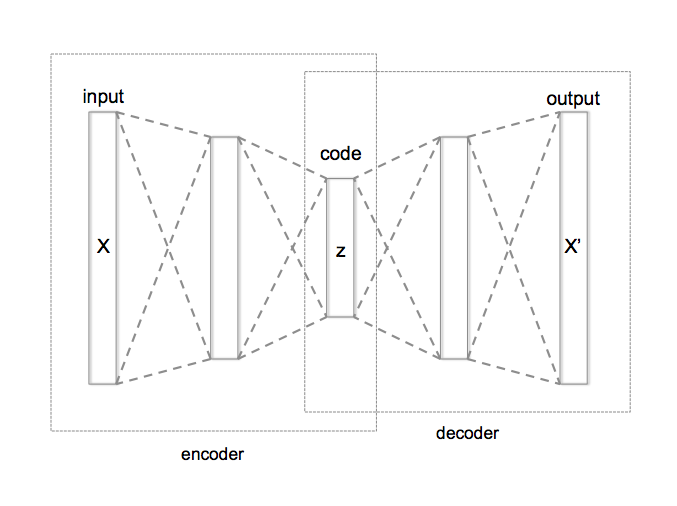## Denoise application

Let’s see how one can create a simple convolutional network to encode the information of the handwritten digit following a Francois Chollet’s post on his keras blog. To train the network we use the old classic mnist handwritten digits dataset (28x28x1 gray-scale images of handwritten digits):

``````from keras.datasets import mnist
(x_train, _), (x_test, y_test) = mnist.load_data()

x_train = x_train.astype('float32') / 255
x_test = x_test.astype('float32') / 255
``````

here we download the dataset directly from the keras library and we normalize the 0-255 color code.

Since we want to build a noise robust model, we artificially add noise to the input dataset: first we apply a Gaussian filter to each image, and finally, we add white noise. To avoid building a model that corrects only for a particular type/magnitude of noise and blurring, for each image in the dataset, we changed the amount of blurring (randomly modifying the sigma of the Gaussian filter) and the magnitude of the white noise. Moreover, we perform soft data augmentation by creating four copies of the training data (exploiting the fact that, during the training, the noise introduced in each image will be different).

``````import numpy as np
from scipy import ndimage

x_train = np.concatenate((x_train, x_train, x_train, x_train), axis=0)
x_test = np.concatenate((x_test, x_test, x_test, x_test), axis=0)

x_train_noise = np.copy(x_train)
for x in x_train_noise:
blurring = np.random.uniform(0,2)
x[...] = ndimage.gaussian_filter(x.reshape(28, 28), sigma=blurring).flatten()
rnd_range = np.random.uniform(0.01,1.5)
x[...] = x[...] + np.random.uniform(-rnd_range, rnd_range, size=x[...].shape)

x_train_2d = x_train.reshape(x_train.shape, 28, 28, 1)
x_train_2d_noise = x_train_noise.reshape(x_train_noise.shape, 28, 28, 1)

x_test_2d = x_test.reshape(x_test.shape, 28, 28, 1)
``````

Note that we reshaped the training (and test) dataset to match `(x_train.shape, 28, 28, 1)` to follow the common convention used in a convolutional network in with the shape of the dataset is given by: (number_of_samples, x_pixels, y_pixels, color_channel). Since here we deal with gray-scale images, we just need one color channel.

The final training dataset has 240000 samples.

### Autoencoder model

We define the autoencoder model as follow:

``````from keras.layers import Input, Dense

input_img = Input(shape=(28, 28, 1))

x = Conv2D(16, (3, 3), activation='relu', padding='same')(input_img)
x = Conv2D(8, (3, 3), activation='relu', padding='same')(x)
x = Conv2D(8, (3, 3), activation='relu', padding='same')(x)

x = Conv2D(8, (3, 3), activation='relu', padding='same')(encoded)
x = UpSampling2D((2, 2))(x)
x = Conv2D(8, (3, 3), activation='relu', padding='same')(x)
x = UpSampling2D((2, 2))(x)
x = Conv2D(16, (3, 3), activation='relu')(x)
x = UpSampling2D((2, 2))(x)
decoded = Conv2D(1, (3, 3), activation='sigmoid', padding='same')(x)
``````

We notice how the model is almost symmetric with respect to the middle layer (the encoded layer). The input (and output) size of the data is `784 (28*28)`, while the encoded representation size is (`128 (4*4*8)`), which is about 6 times smaller. The network is not completely symmetric because of the `MaxPooling2D` vs `UpSampling2D` layers. These two layers have no trainable parameters but they are only used to deterministically decrease (down-sampling) and increase (up-sampling) the dimension of a 2D matrix. In our example MaxPooling split the input matrix into (2x2) regions, and replaces the region with the maximum between the 4 values contained in it, shrinking the original matrix size by a factor of four. This is a convolutional operation and cannot be mathematically inverted, therefore the neural network cannot be completely symmetric. We can approximate the inversion of `MaxPooling2D` in different ways: one is to replace each pixel of the input image with 4 copy of it (`UpSampling2D`) and the second is to make the model learn the deconvolution operation as well (`Conv2DTranspose`). The latter option can lead to better performance but it is computationally heavier. Here, we choose to use the `UpSampling2D` method for its simplicity.

### Model training

It is time to train the model. Following again Francois Chollet’s suggestion, we used the `adadelta` optimizer and `binary_crossentropy` as losses. To train the network we exploited the GPU runtime of (Google Colab)[colab.research.google.com], which gave us a performance boost of almost a factor of 40 (from about 309 seconds per epoch to 8 seconds).

``````from keras.models import Model, load_model
from keras.layers import Conv2D, MaxPooling2D, UpSampling2D

autoencoder = Model(input_img, decoded)
​
out = autoencoder.fit(x_train_2d_noise, x_train_2d,
epochs=500,
batch_size=256,
shuffle=True,
validation_data=(x_test_2d, x_test_2d),
verbose=1)
``````

After 500 epochs of training the loss function seems to be saturated, without showing any overfitting issue: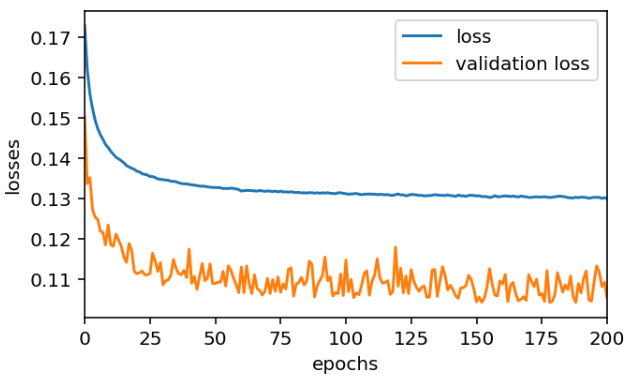Note how, apparently surprisingly, the validation loss seems lower than the training loss. This happens because the validation dataset is noise-free and therefore is much easier to encode.

### Testing the model

Let’s test how the model performs on the test image dataset: we pick a random image and we feed it into the autoencoder network by progressively increasing the blurring and the noise level:

``````import matplotlib.pyplot as plt
fig, [ax_input, ax_output] = plt.subplots(2, 6, figsize=(12, 4))

for i in range(6):
ax_input[i].set_xticks([])
ax_input[i].set_yticks([])
ax_output[i].set_xticks([])
ax_output[i].set_yticks([])

input_image = x_test_2d.reshape(28, 28)
input_image = ndimage.gaussian_filter(input_image, sigma=0.5*i)
white_noise = np.random.uniform(-0.2*i,0.2*i, size=input_image.shape) * 2
input_image = input_image + white_noise

ax_input[i].imshow(input_image, cmap='gray')
ax_input[i].set_title(f's={0.5*i:.1f}, r={0.2*i:.1f}')

output_image = autoencoder.predict(input_image.reshape(1, 28, 28, 1))
ax_output[i].imshow(output_image.reshape(28, 28), cmap='gray')
``````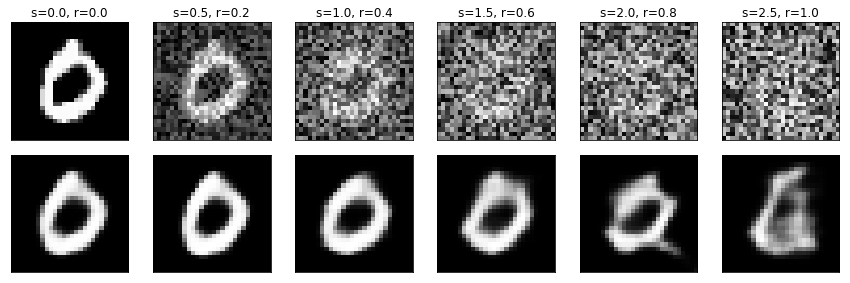As we can see the model can drastically reduce the noise in the image, showing good results even for very high noise levels, when the digit corresponding to the input image is so blurred and noisy it cannot be identified even by humans.

Here we plot a second example with high blurring and noise for different digits, C.S.I. yet?